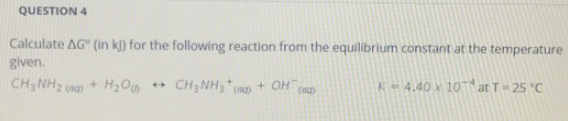# Problem: Calculate ΔG° (in kJ) for the following reaction from the equilibrium constant at the temperature given. CH3NH2 (aq) + H2O (l) &lt;-&gt; CH3NH3+ (aq) + OH - (aq)      K = 4.40 x 10 -4 at T = 25°C

###### FREE Expert Solution
90% (489 ratings)###### Problem Details

Calculate ΔG° (in kJ) for the following reaction from the equilibrium constant at the temperature given.

CH3NH2 (aq) + H2O (l) <-> CH3NH3+ (aq) + OH - (aq)      K = 4.40 x 10 -4 at T = 25°C